Optimization using Python: Linear Programming with example

By: Ram on Nov 04, 2020

In the scenario, there are 64 different foods and their various nutrient contents and calories.

Also, the price of each of these food items. We can want to formulate this as a diet optimization problem and find the optimal size of the food intake.

A typical optimization scenario will have an objective function (Min/Max), a list of decision variables, and constraints.

In the diet scenario, we will try to define each of these.

Objective:

Minimize the cost of the diet (Price per serving * Quantity of serving for each item)

Parameters

Cost per unit (serving) for each of the food items

Decision Variables

Quantity of Food Items (serving quantity)

Constraints

Minimum nutrients required

Maximum nutrients allowed

Each of the decision variables is non-negative

Data and Parameters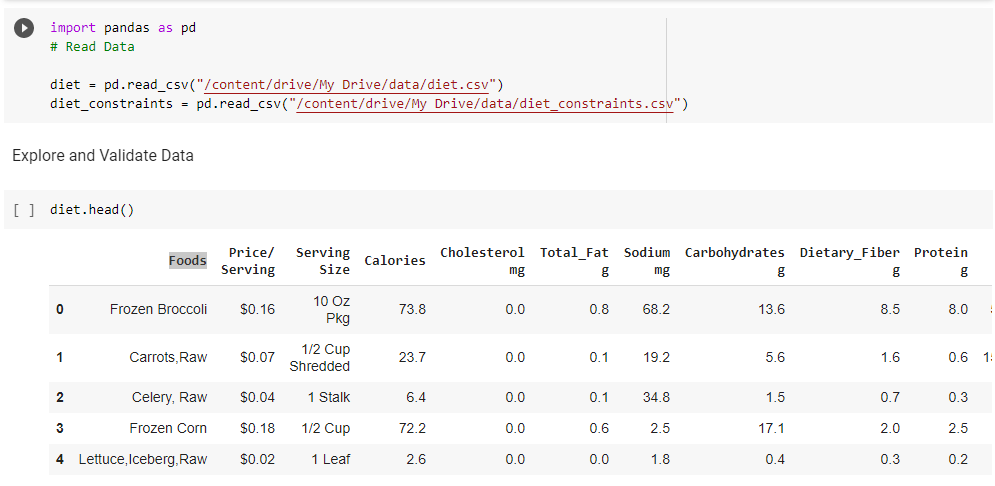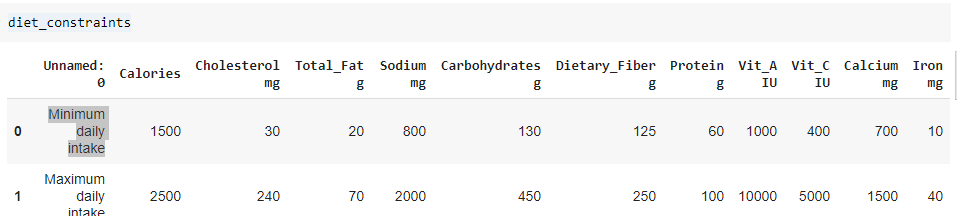diet_constraints.loc['Minimum daily intake']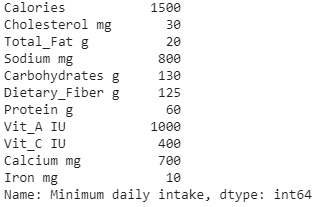# Define Index

diet_constraints.index=diet_constraints['Unnamed: 0'].values

# Drop column

diet_constraints=diet_constraints.drop('Unnamed: 0', axis=1)

# View Data Frame

diet_constraints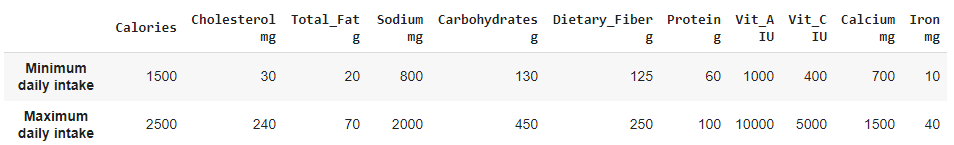Find the number of food items in the data frame

diet.shape

(64, 14)

It has 64 food items with each row for it.

diet.columns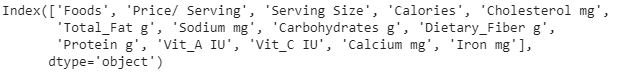It has 14 columns, the first 3 indicate the food item name, the price for a serving, and serving size. From the calories onward, it has information on nutrients contents in each of these food items.

Model Formulation

Before solving an optimization problem, we need to formulate the problem based on the context information available.

For this scenario, we have a list of food items, the price per serving for each of the food, and nutrients information. Also, allowable minimum and maximum nutrients information are available.

To start with, we are formulating this as a Linear Programming problem with an objective to find the combination of food items and their serving quantity (optimal) which minimize the cost but satisfy the nutrient requirements (both minimum and maximum).

Objective Function

Z (Min)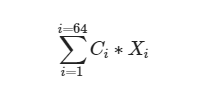Decision Variables

Xi: Quantity of Food i to be given

There are 64 different types of food available for selection.

Parameters

Nij: Nutrient j in the food i

Ci: Cost of Food i for serving unit

Constraints

1. The minimum value of nutrients and calories to be consumed.

Pj: Minimum Value for Nutrient/Calorie required

Sum of Xi * Nij >= Pj or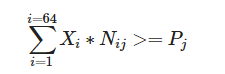for each value of j

1. Maximum nutrients and calories allowed

Rj: Maximum Value for Nutrient/Calorie Allowed

Sum of Xi * Nij <= Rj or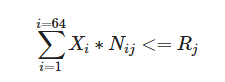for each value of j

1. Non negative decision variables Xi >=0

Linear Programming Optimization: Python Set Up

We are using the PuLP package in Python to solve this scenario. There are a few other options such as Gurobi, and CPLEX.

Install the package

! pip install pulp

Now, we need to create an optimization object to add optimization details to this object.

# Define Model object Type

import pulp as plp

diet_lp_model = plp.LpProblem(name="DietLPModel")

Now, the Model Object is named as diet_lp_model and has been created. Next, we need to create decision variables.

A Python dictionary (or Pandas Series) can be created where dictionary keys are decision variables, and values are decision variable objects.

This is a scenario of Linear Programming, so the decision variables are continuous. In Mix Integer Programming, the decision variables can also be integer or binary. We will try the Mix Integer Programming based model as well.

Assign Parameters

We have prices per serving for each of the food items, calories, and nutrients information for each of the food items, the minimum and maximum allowed quantities for the constraints. We are creating dictionaries for each of these parameters.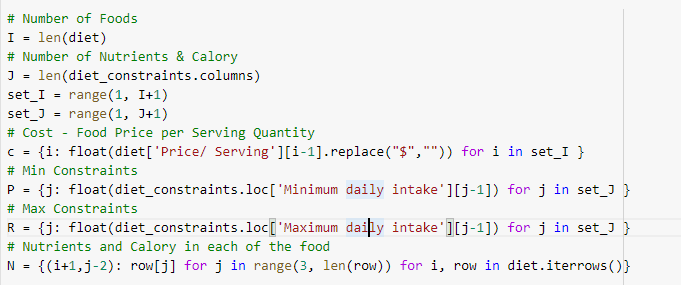We also need to define the lower and upper bounds for each of the decision variables. In this scenario, all the decision variables should be non-negative.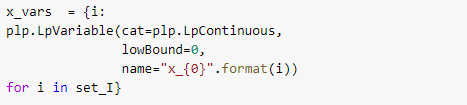Constraints

Setting minimum and maximum value constraints.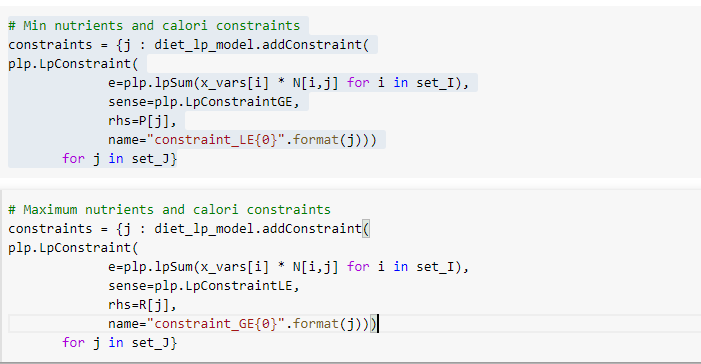These constraints are added to the model object created earlier.

Objective

Now, we need to add an objective function to this object.  This is a cost minimization problem.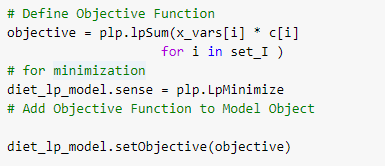Now, we have added all the elements of optimization – objective function, constraints, and decision variables. We are ready to submit the model object for the solution.

We can validate the details by print the optimization object.

Solve Optimization

There are a few solver options. But we are using the default option which is CBC.

# solving with CBC

sol =diet_lp_model.solve()

pulp.LpStatus[sol]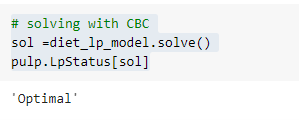Now we can view the optimal values for each of the decision variables.

opt_df = pd.DataFrame.from_dict(x_vars, orient="index", columns = ["variable_object"])

opt_df["solution_value"] = opt_df["variable_object"].apply(lambda item: item.varValue)

Optimal Solution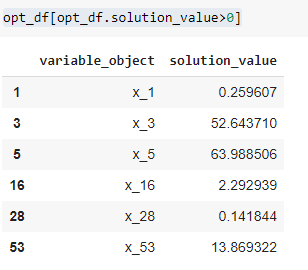Food items for optimal Solutions.

Keep in the mind the index values. The Diet data frame has index values starting from 0 whereas the Optimal Solution index starts with 1.

We need to do index adjustment before merging.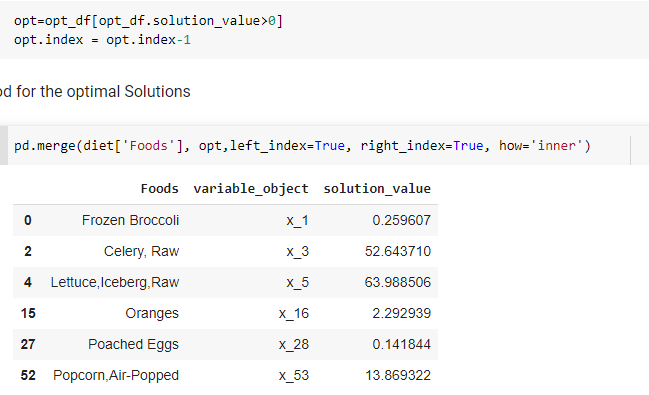Tags
Most PopularJun 18, 2020Jul 23, 2020Jun 19, 2020Jun 19, 2021